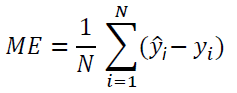# Linear Regression for Data Science

In this article, we discuss the importance of linear regression in data science and machine learning.Image by Author

## Key Takeaways

• Most beginners interested in getting into the field of data science are always concerned about the math requirements.
• Data science is a very quantitative field that requires advanced mathematics.
• But to get started, you only need to master a few math topics.
• In this article, we discuss the importance of linear regression in data science and machine learning.

# Linear Regression (Continuous Target Variable)

Regression models are the most popular machine learning models. Regression models are used for predicting target variables on a continuous scale. Regression models find applications in almost every field of study, and as a result, it is one of the most widely used machine learning models. This article will discuss the basics of linear regression and is intended for beginners in the field of data science.

## 1. Simple Linear Regression

In simple linear regression, there is only one predictor variable. Since our goal is to predict the crew variable, we see from Figure 1 that the cabins variable correlates the most with the crew variable. Hence our simple regression model can be expressed in the form:where m is the slope or regression coefficient, and c is the intercept

## 2. Multiple Linear Regression

Suppose that the target variable now depends on several predictor variables (for e.g. four predictor variables), then the system can be modeled using multiple regression analysis:where X is the features matrix, w_0 is the intercept, and w_1, w_2, w_3, and w_4 are the regression coefficients.

# Evaluating Linear Regression Models

The most popular metric used for evaluating the performance of linear regression models is the R2 score metric which can be calculated as follows:The R2 score takes values between 0 and 1. When R2 is close to 1, it means the predicted values agree closely with the actual values. If R2 is close to zero, then it means the predictive power of the model is very poor.

Other metrics that could also be used for evaluating a linear regression model include the following:

MSE (Mean Square Error): Uses Euclidean distance to calculate the error. MSE gives the magnitude of the error only.MAE (Mean Absolute Error): Uses Manhattan distance to calculate the error. MAE (like MSE) gives the magnitude of the error only.ME (Mean Error): Keeps track of the sign of error, is model over-predicting (ME > 0) or under-predicting (ME < 0)?• R2 Score is a very popular metric used for evaluating the performance of linear regression models.
• Use MSE or MAE when comparing two or more models, the lower the value of MSE or MAE, the better.
• Use ME when you are interested in understanding if on average, your model is over-predicting (ME > 0) or under-predicting (ME < 0). One could also use the R2 Score to compare different models.

# Case Study: Machine Learning Model for Predicting Ship Crew Size

In this case study, we build a multiple linear regression model using the cruise_ship_info.csv dataset for predicting a ship's crew size. The dataset and code for this project can be downloaded from this GitHub repository: https://github.com/bot13956/ML_Model_for_Predicting_Ships_Crew_Size

# Summary

• Linear regression (for continuous target variable prediction) is the most popular machine learning model. Regression models find applications in almost every field of study, and as a result, it is one of the most widely used machine learning models.
• Linear regression models can be classified into simple regression (single feature) and multiple regression (several target variables) models.
• Linear regression models can be implemented using software libraries such as Pylab, Numpy, or scikit-learn.
• Several metrics can be used for evaluating regression models such as MSE, ME, MAE, and R2 Score. The R2 score remains the most popular metric.
• Other regression models are KNR (k-neighbors regression) and SVR (support vector regression).

Benjamin O. Tayo is a Physicist, Data Science Educator, and Writer, as well as the Owner of DataScienceHub. Previously, Benjamin was teaching Engineering and Physics at U. of Central Oklahoma, Grand Canyon U., and Pittsburgh State U.